# The QUANTLIFE Procedure (Experimental)

### Kaplan-Meier-Type Estimator for Censored Quantile Regression

Portnoy (2003) proposes the use of weighted quantile regression to sequentially estimate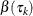along the equally spaced grid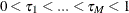. You can request this method by specifying the METHOD=KM option in the PROC QUANTLIFE statement. The grid pointsare equally spaced with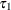specified by the INITTAU= option and the step between adjacent grid points specified by the GRIDSIZE=option.

This method uses a weight function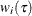for each censored observation. The weight function is constructed as follows: Let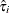be the first grid point at which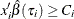and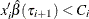; otherwise let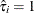. When computing theth quantile, assign weight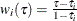to the censored observation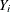if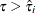; otherwise assign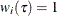. The algorithm for computing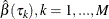is as follows:

1. Compute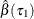by using the standard quantile regression method.

2. For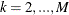, obtain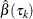sequentially by minimizing the following weighted quantile regression objective function: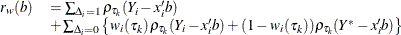where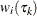is the weight for the right-censored observationat computing, and the complementary weight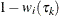are for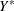, a large constant that is greater than all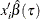.

The weighted quantile regression method is similar to Efron’s redistribution-of-mass idea (Efron, 1967) for the Kaplan-Meier estimator.

Note that if all observations are uncensored,is the same as the standard quantile regression estimator.